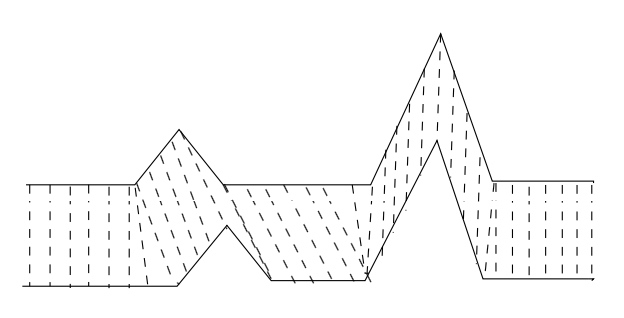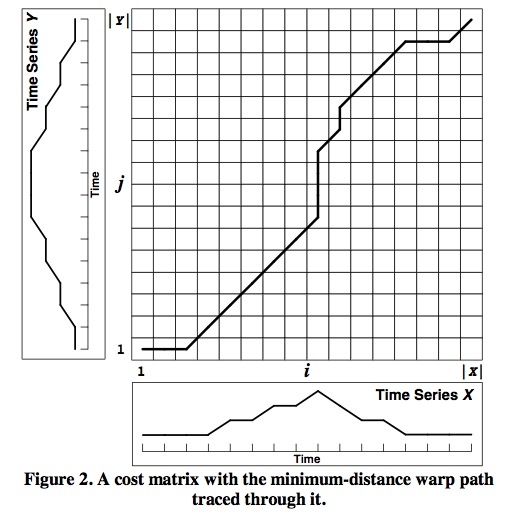DTW 算法用于解决语音序列不对齐的问题。因为不同的人发言速度不一样，所以很难直接比对两个序列的相似性。## 问题转化• 边界条件：$$w_1=(1,1)$$$$w_k=(m, n)$$。首尾需要对齐
• 连续性和单调性：如果 $$w_{k-1}=(a',b')$$，那么对于路径的下一个点 $$w_{k}=(a,b)$$ 需要满足 $$0 \le a-a' \le 1$$$$0 \le b-b' \le 1$$

\begin{aligned} D(i,j)&=Dist(i,j)+\min(D(i-1,j),D(i,j-1),D(i-1,j-1)) \\ D(1,1)&=0 \end{aligned}